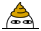# BZOJ-3875: [Ahoi2014&Jsoi2014]骑士游戏

[文章目录]

### Description

JYY一共有两种攻击方式，一种是普通攻击，一种是法术攻击。两种攻击方式都会消耗JYY一些体力。采用普通攻击进攻怪兽并不能把怪兽彻底杀死，怪兽的尸体可以变出其他一些新的怪兽，注意一个怪兽可能经过若干次普通攻击后变回一个或更多同样的怪兽；而采用法术攻击则可以彻底将一个怪兽杀死。游戏世界中一共有N种不同的怪兽，分别由1到N编号，现在1号怪兽入侵村庄了，JYY想知道，最少花费多少体力值才能将所有村庄中的怪兽全部杀死呢？2<=N<=2*10^5,1<=Ri,Sigma(Ri)<=10^6,1<=Ki,Si<=5*10^14

#include <queue>
#include <vector>
#include <cstdio>
#include <cstring>
#include <algorithm>
using namespace std;
typedef long long ll;
ll dis,p;
int n,rd;
vector<int>v,v1;
bool vis;
{
x=0; int f=1; char ch=getchar();
while(ch<'0'||ch>'9') {if(ch=='-') f=-1; ch=getchar();}
while(ch>='0'&&ch<='9') x=x*10+ch-'0',ch=getchar(); x*=f;
}
{
x=0; int f=1; char ch=getchar();
while(ch<'0'||ch>'9') {if(ch=='-') f=-1; ch=getchar();}
while(ch>='0'&&ch<='9') x=x*10+ch-'0',ch=getchar(); x*=f;
}
struct node
{
int v;ll dis;
};
bool operator < (node x,node y) {return x.dis>y.dis;}
priority_queue<node>he;
int main()
{
for(int i=1;i<=n;++i)
{
for(int j=1;j<=rd[i];++j)
{
v1[i].push_back(x);
}
if(!rd[i]) dis[i]=min(dis[i],p[i]);
}
for(int i=1;i<=n;++i) he.push((node){i,dis[i]});
for(int i=1;i<=n;++i)
{
while(!he.empty())
{
x=he.top().v; he.pop();
if(vis[x]) continue; else break;
}
vis[x]=1;
for(int j=0;j!=v[x].size();++j)
{
y=v[x][j]; rd[y]--;
if(!rd[y]&&!vis[y])
{
ll tmp=p[y];
for(int k=0;k!=v1[y].size();++k) tmp+=dis[v1[y][k]];
if(tmp<dis[y]) dis[y]=tmp,he.push((node){y,dis[y]});
}
}
}
printf("%lld",dis);
return 0;
}


#### 3 条评论

1.张翼德说道：

bool operator < (node x,node y) {return x.dis>y.dis;}
这里为什么是大于号啊？？1.Lijin说道：

@张翼德 QwQ，抱歉，之前没看到。其实那个是小根堆的。

2.张翼德说道：

您的堆为什么是大根堆啊？？
地杰斯特拉不是每次找距离联通块最小的点嘛？QWQ